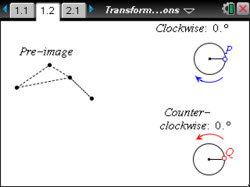# Activities

••• ##### Subject Area

• Math: Geometry: Transformational Geometry

• ##### Author9-12

45 Minutes

• ##### Device
•TI-Nspire™ CX/CX II
•TI-Nspire™ CX CAS/CX II CAS
• TI-Nspire™ Navigator™
• TI-Nspire™
• TI-Nspire™ CAS
• ##### Software

TI-Nspire™
TI-Nspire™ CAS

3.0

• ##### Report an Issue

Transformations: Rotations#### Activity Overview

Explore clockwise and counterclockwise rotations to discover the properties of the pre-image and image of a triangle.

#### Objectives

• Students will identify a rotation as an isometry, also called a congruence transformation.
• Students will identify which properties (side length, angle measure, perimeter, area, and orientation) of a figure are preserved in a rotation and which are not.
• Students will describe the relationship between the sign of the angle and the direction of rotation.
• Students will identify coordinates of an image that is rotated about the origin through angles of ±90°, ±180°, ±270°, and ±360°.
• Students will generalize the relationship between the coordinates of a pre-image and its image in a rotation about the origin in the coordinate plane.
• Look for and make use of structure. (CCSS Mathematical Practice)
• Look for and express regularity in repeated reasoning. (CCSS Mathematical Practice)

#### Vocabulary

• pre-image
• image
• transformation
• rotation
• clockwise / counterclockwise direction
• congruent figures
• congruence transformation
• isometry
• positive and negative angles